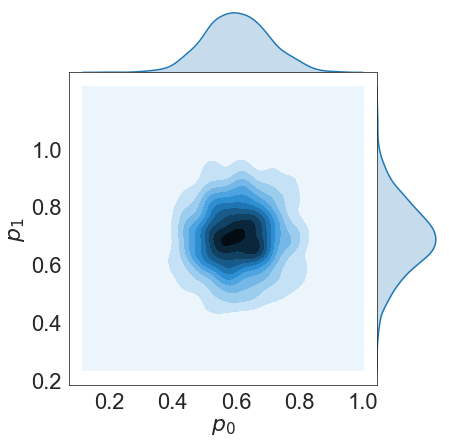# Joint Distributions¶

Author(s) Paul Miles | August 7, 2018

This tutorial provides a demonstration of the joint distributions plotting routine available in mcmcplot.

In :
# import required packages
import numpy as np
from mcmcplot import mcseaborn as mcs
import warnings
warnings.filterwarnings("ignore")


### Generate Random Chains¶

The plotting routines are designed to be used in conjunction with the result of a MCMC simulation. For the purpose of this example, we consider a randomly generated chain. We will consider a chain with 3 parameters that have the following distributions:

• $p_{0} \sim N(1.0, 0.5)$
• $p_{1} \sim N(2.5, 3.0)$
• $p_{2} \sim N(-1.3, 0.75)$
In :
nsimu = 1000
npar = 3
mu = np.array([1.0, 2.5, -1.3])
sig = np.array([0.5, 3.0, 0.75])
chain = np.zeros([nsimu, npar])
for ii in range(npar):
chain[:,ii] = sig[ii]*np.random.randn(nsimu,) + mu[ii]


### Plot Default Joint Distributions¶

This routine will generate a joint distribution plot for each parameter combination in the chain. For this example, npar = 3, so 3 different plots will be generated representing the combinations:

• $p_0$, $p_1$
• $p_0$, $p_2$
• $p_1$, $p_2$

Note, that each function call returns the figure handles as well as the settings used to generate the plots.

In :
fjd, used_settings = mcs.plot_joint_distributions(chains = chain, settings = None)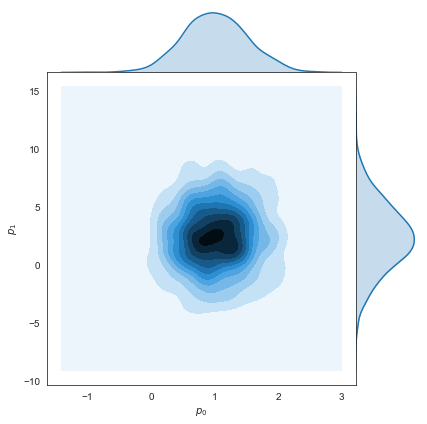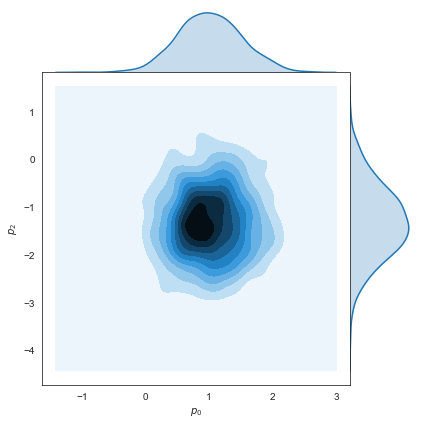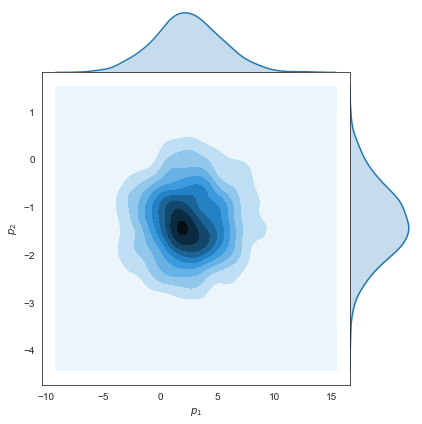### Change the Type of Joint Plot, Figure Size, and Color¶

We can easily adjust the type of joint plot, size of the figure, and color map defining the following settings.

In :
settings = {'jointplot': {'kind': 'hex', 'height': 4, 'color': 'g'}}
fjd, used_settings = mcs.plot_joint_distributions(chains = chain[:,0:2], settings = settings)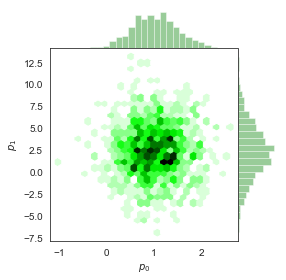In :
settings = {'jointplot': {'kind': 'reg', 'height': 6, 'color': 'b'}}
fjd, used_settings = mcs.plot_joint_distributions(chains = chain[:,0:2], settings = settings)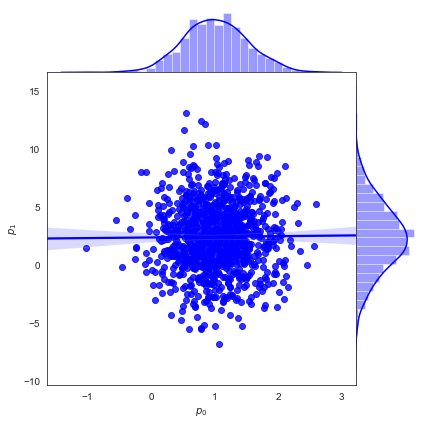### Edit the seaborn Style¶

The default seaborn style is white, but it can be changed by defining the sns_style variable. Each style has it's own settings, so see the seaborn style documentation for details.

In :
settings = {'jointplot': {'kind': 'scatter', 'height': 4, 'color': 'k'}}
fjd, used_settings = mcs.plot_joint_distributions(chains = chain[:,0:2], sns_style='dark', settings = settings)

settings = {'sns': {'figure.facecolor': 'yellow'}}
fjd, used_settings = mcs.plot_joint_distributions(chains = chain[:,0:2], settings = settings)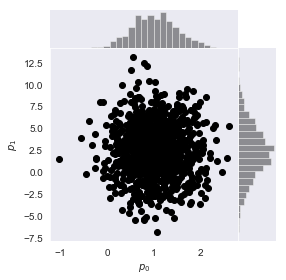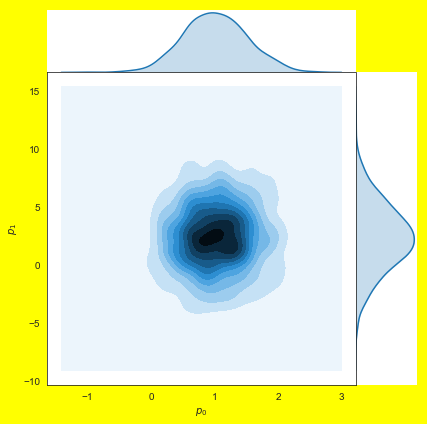### Manually Edit Features¶

As seaborn is built on top of matplotlib, you can edit various plot features in the same way. The output from the plot_joint_distributions method will contain the figure handles inside a list.

In :
fjd, used_settings = mcs.plot_joint_distributions(chains = chain[:,0:2], settings = None)
a = fjd.ax_joint;
a.set_xticklabels(a.get_xticklabels(), fontsize = 22);
a.set_yticklabels(a.get_yticklabels(), fontsize = 22);
a.set_xlabel(a.get_xlabel(), fontsize = 22);
a.set_ylabel(a.get_ylabel(), fontsize = 22);
f = fjd
f.savefig('basic_joint_distributions.png')